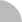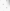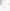# 臺灣博碩士論文加值系統

(3.238.204.167) 您好！臺灣時間：2022/08/09 21:20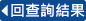:::

### 詳目顯示

: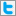Twitter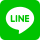•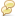被引用:0
•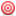點閱:116
•評分: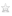•下載:6
•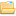書目收藏:0
 給定一個圖形G， G的L(d_{1},d_{2};p,q)-labeling是指一個函數 f:V(G)-->{0,1,2,...,n}使得：在d_{G}(u,v)<=d_{1}的情況下，則 |f(u)-f(v)|>=p；而且，在 d_{G}(u,v)<=d_{2}的情況下，則|f(u)-f(v)|>=q。一個k-L(d_{1},d_{2};p,q)-labeling是指一個 L(d_{1},d_{2};p,q)-labeling f使得 max_{vin V(G)}f(v)<=k。我們把圖形G的L(d_{1},d_{2};p,q)-labeling number,記成符號lambda_{d_{1},d_{2}}^{p,q}(G),是指使得圖形G有一個k-L(d_{1},d_{2};p,q)-labeling的最小數k。在這篇論文中，我們對於一般的圖形和一些特殊的圖形給了L(d_{1},d_{2};p,q)-labeling number的上界和下界 。當圖形G是一條path，我們也討論圖形G的L(d_{1},d_{2};p,q)-labeling number
 Given a graph G, an L(d_{1},d_{2};p,q)-labeling of G is a function f:V(G)-->{0,1,2,...,n} such that |f(u)-f(v)|>= p if d_{G}(u,v)<= d_{1} and|f(u)-f(v)|>= q if d_{G}(u,v)<= d_{2}. A k-L(d_{1},d_{2};p,q)-labeling is an L(d_{1},d_{2};p,q)-labeling f such that max_{vin V(G)}f(v)<= k.The L(d_{1},d_{2};p,q)-labeling number of G, denoted by lambda_{d_{1},d_{2}}^{p,q}(G), is the smallest number k such that G has a k-L(d_{1},d_{2};p,q)-labeling.In this thesis, we give the upper bound and lower bound of the L(d_{1},d_{2};p,q)-labeling number for general graphs and some special graphs. We also discuss L(d_{1},d_{2};p,q)-labeling number of G, when G is a paths.
 1.Introduction2.Upper Bound and Lower Bound of the L(d_{1},d_{2};p,q)-labeling Number of Graphs3.The L(d_{1},d_{2};p,q)-labeling Number of Paths4.Conclusion
  G. J. Chang, W. -T. Ke, D. Kuo, D. D. -F. Liu andR. K. Yeh, ``On L(d,1)-labelings of graphs,'Disc. Math., toappear. G. J. Chang and D. Kuo, ``The L( 2,1)-labelling problem on graphs,'SIAM J. Disc. Math. 9 (1996) 309-316. J. P. Georges and D. W. Mauro, ``Generalized vertex labellings with a condition at distance two,' Conger. Numer.109 (1995) 141-159. J. P. Georges, D. W. Mauro and M. A. Whittlesey,``Relating path covering to vertex labellings with a condition at distance two,' Discrete Math.135 (1994) 103-111. J. R. Grigges and R. K. Yeh, ``Labelling graphswith a condition at distance two,'SIAM J. Disc. Math. 5 (1992)586-595. W. K. Hale, ``Frequency assignment : theory andapplications,' Proc. IEEE, 68 (1980) 1497-1514. J. van den Heuvel, R. A. Leese and M. A. Shepherd,``Graph labelling and radio channel assignment,' J. Graph Theory,to appear. D. Kuo, ``Graph labelling problems,' Ph. D. Thesis,Dept. of Applied Math., National Chiao Tung Univ., Hsinchu, Taiwan (1995). D. D. -F. Liu and R. K. Yeh, ``On distance twolabellings of graphs,'Ars Comb., 47 (1997) 13-22.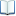電子全文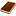國圖紙本論文推文當script無法執行時可按︰推文 網路書籤當script無法執行時可按︰網路書籤 推薦當script無法執行時可按︰推薦 評分當script無法執行時可按︰評分 引用網址當script無法執行時可按︰引用網址 轉寄當script無法執行時可按︰轉寄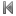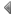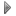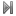top
 無相關論文

 無相關期刊

 1 二元數列之預測方法的模擬比較：預測方法是否需具隨機性？ 2 TTM公開密碼系統 3 層的性質 4 CmXPn和CmXCn的兩起始點傳播問題 5 醫院分類:SVMApproach 6 採集合排序樣本時,常態平均值之較佳檢定 7 積分型式的交叉有效法 8 不可分割成本之等量分配與一致性 9 智慧型天線系統於遠近迴響傳播通道模型之效能評估 10 利用鐵、鎳金屬片及次微米鐵點陣列成長具方向性奈米碳管 11 鎳鐵次微米線的製作與性質研究 12 氧化鈦與氧化釩單層膜與多層膜之製備與特性分析及其光觸媒性質 13 氧化鎢與氧化釩單層膜與多層膜之製備與特性分析及其電致色變性質 14 光纖之破壞分析研究 15 ABAQUS模擬殘留應力對疲勞裂縫成長之研究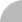簡易查詢 | 進階查詢 | 熱門排行 | 我的研究室